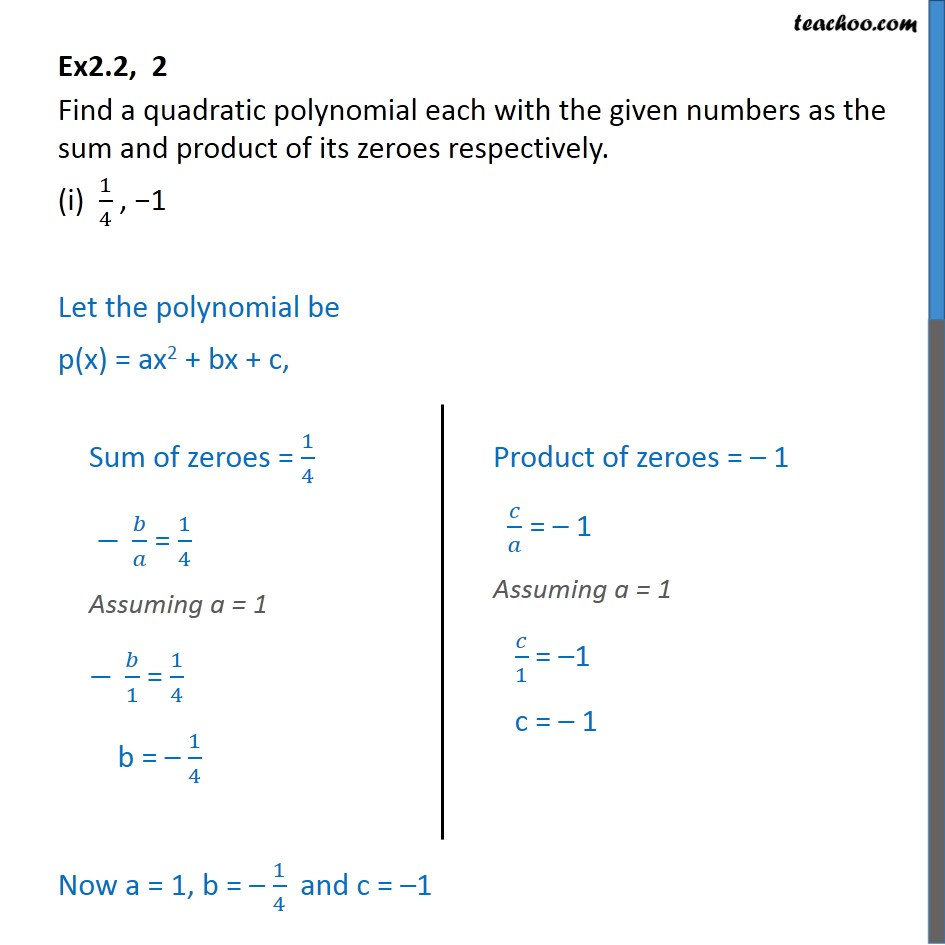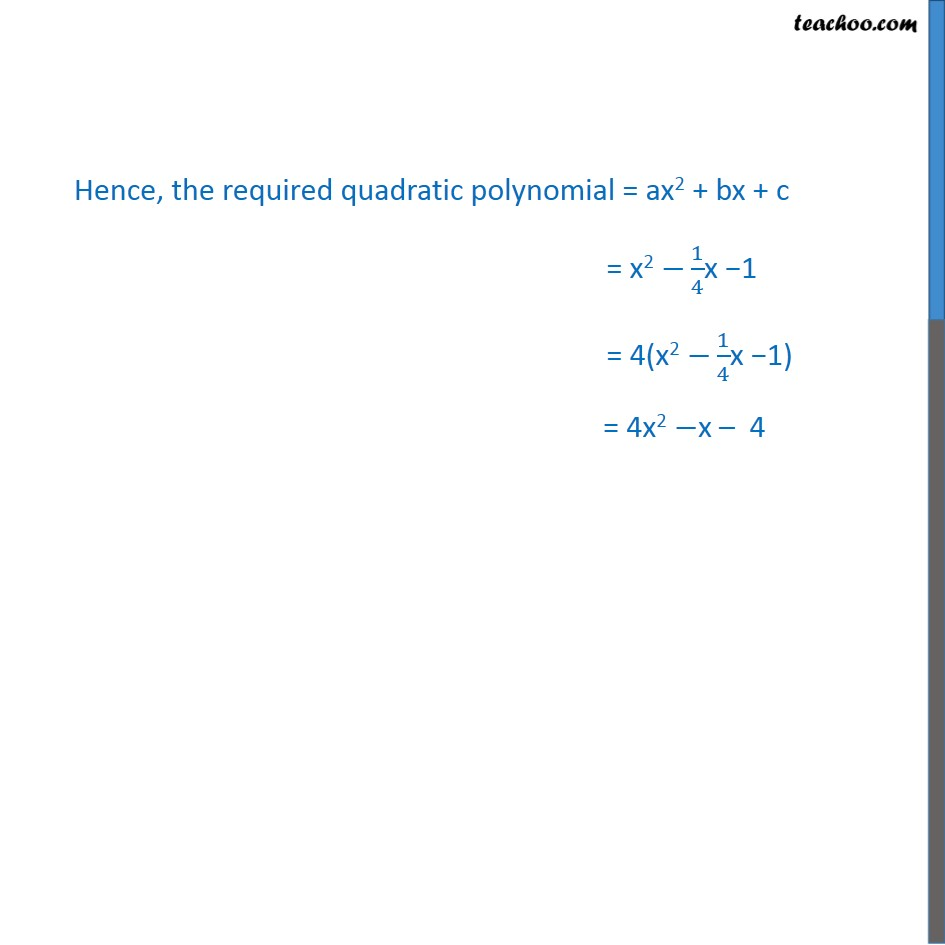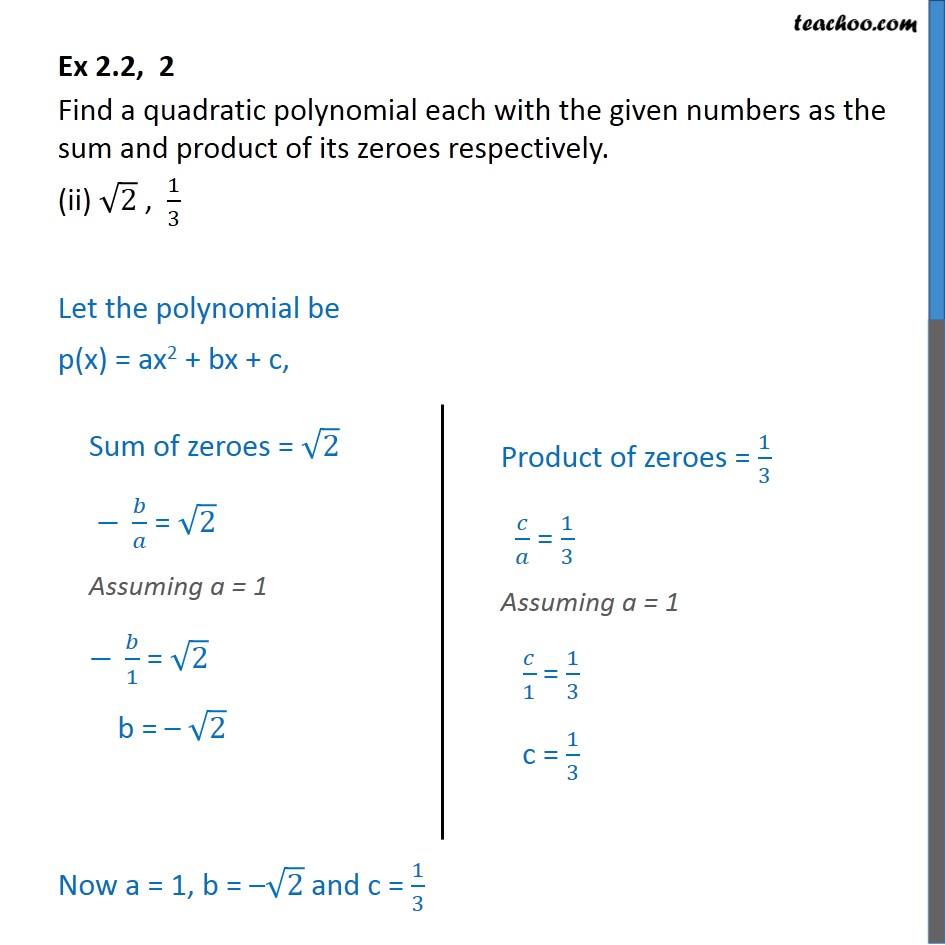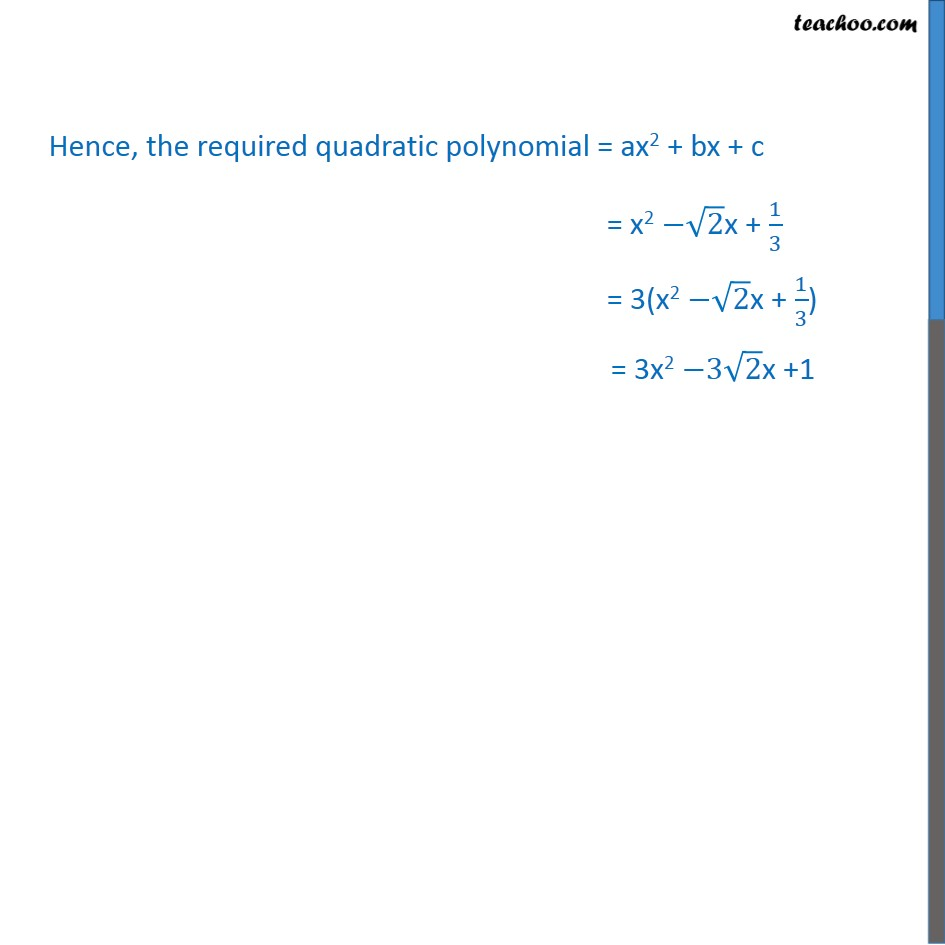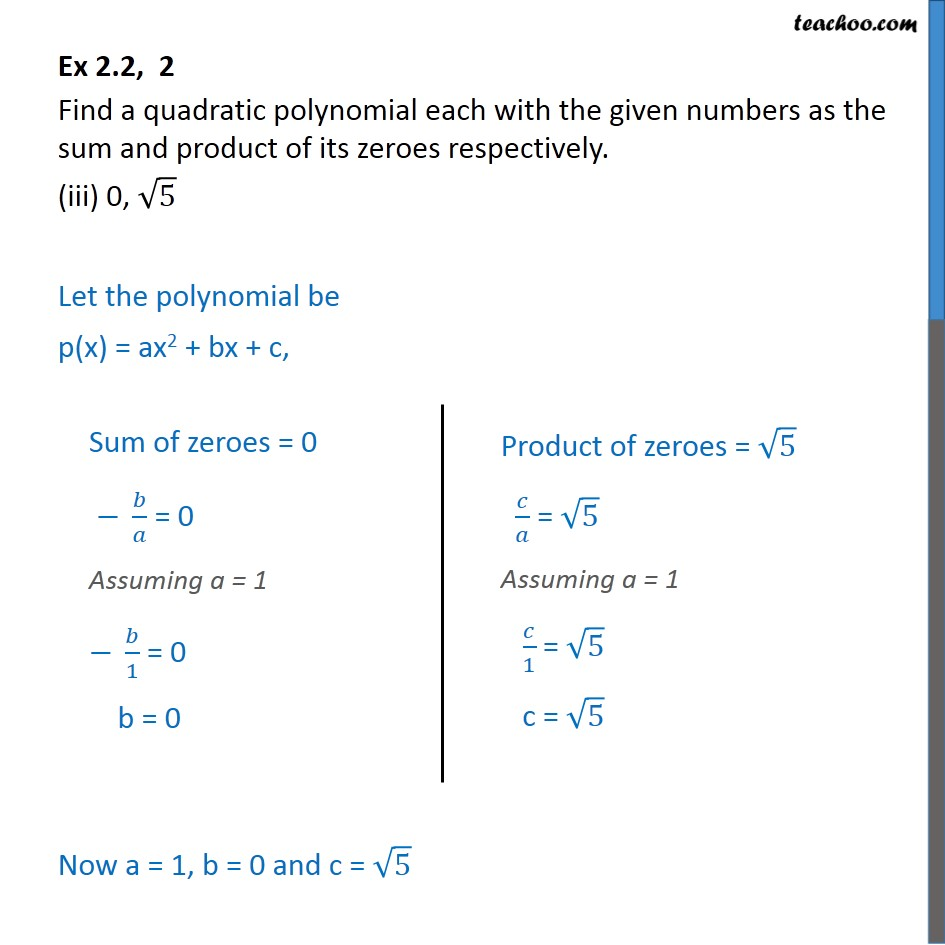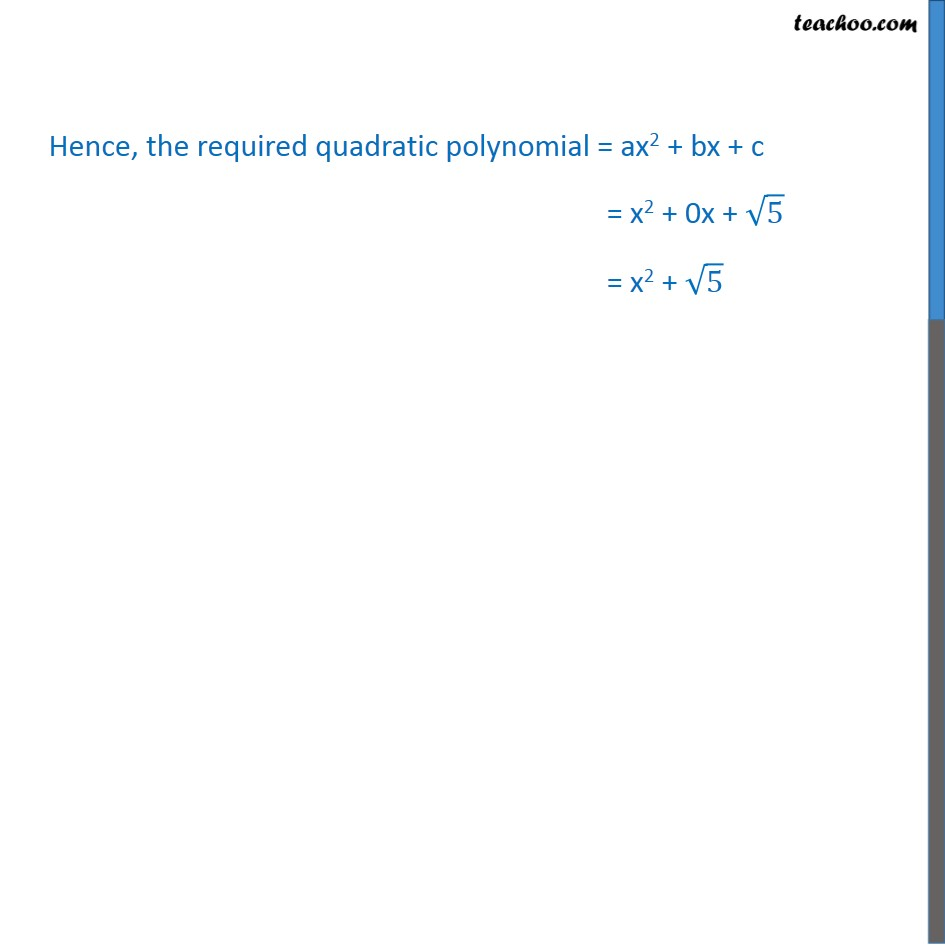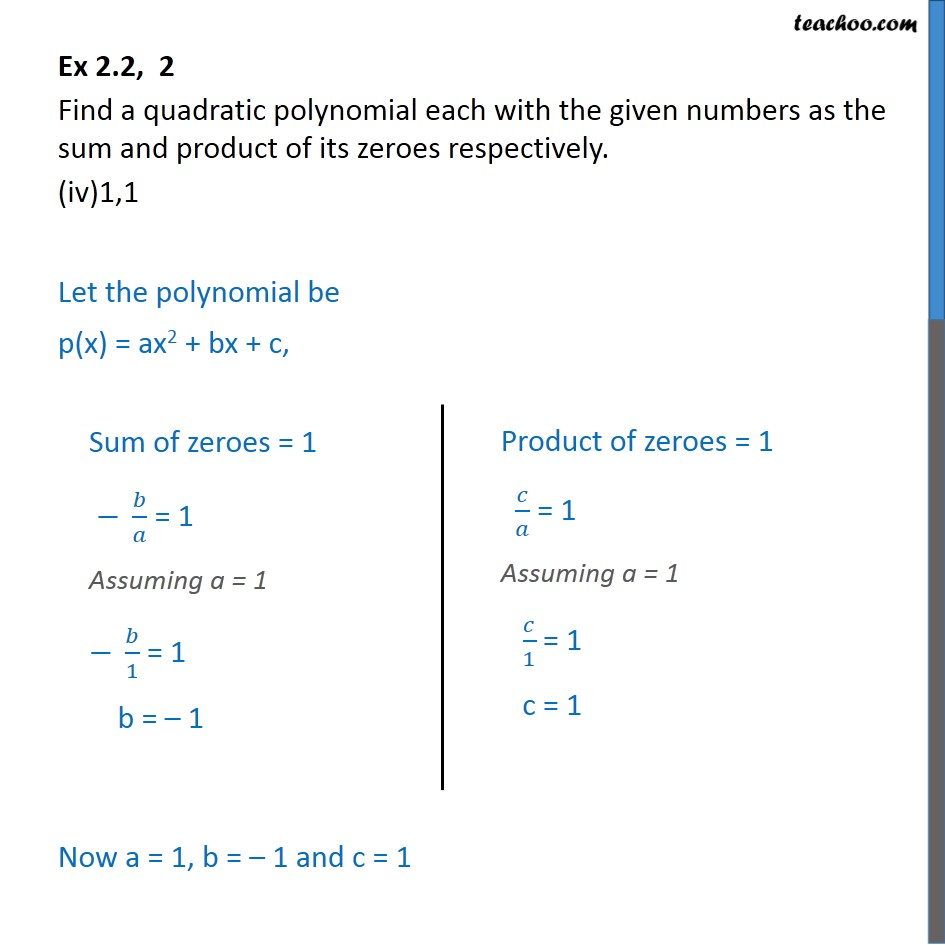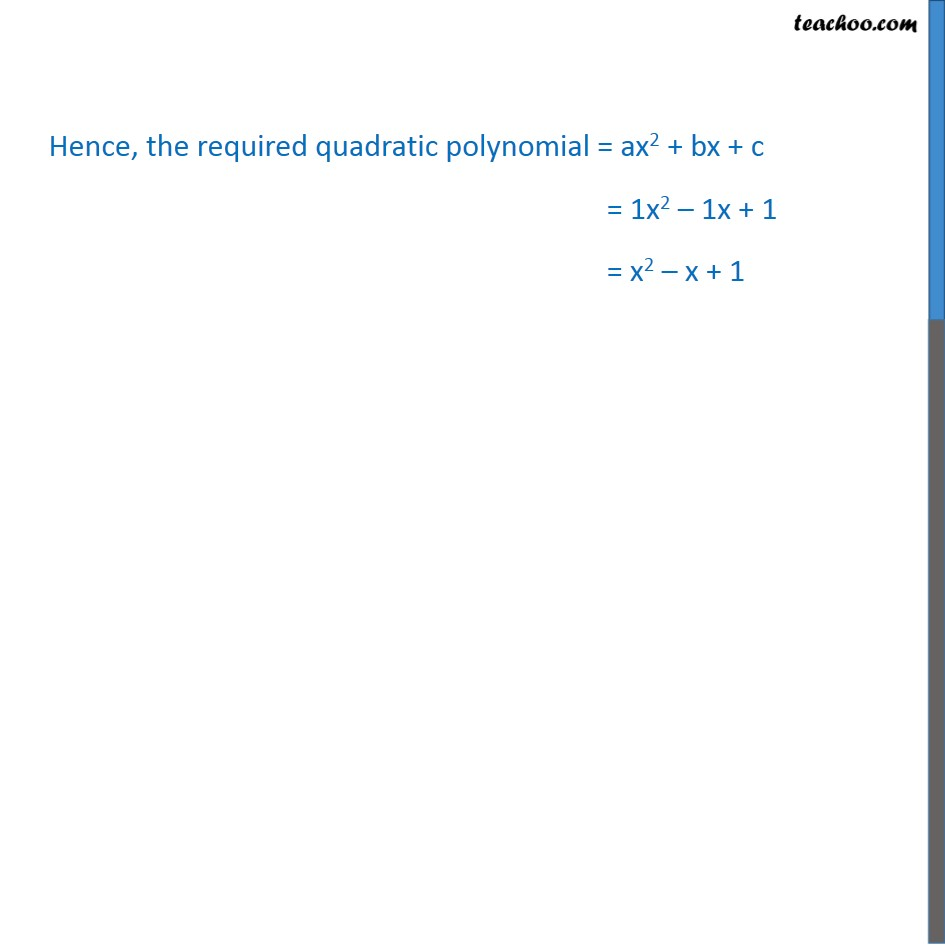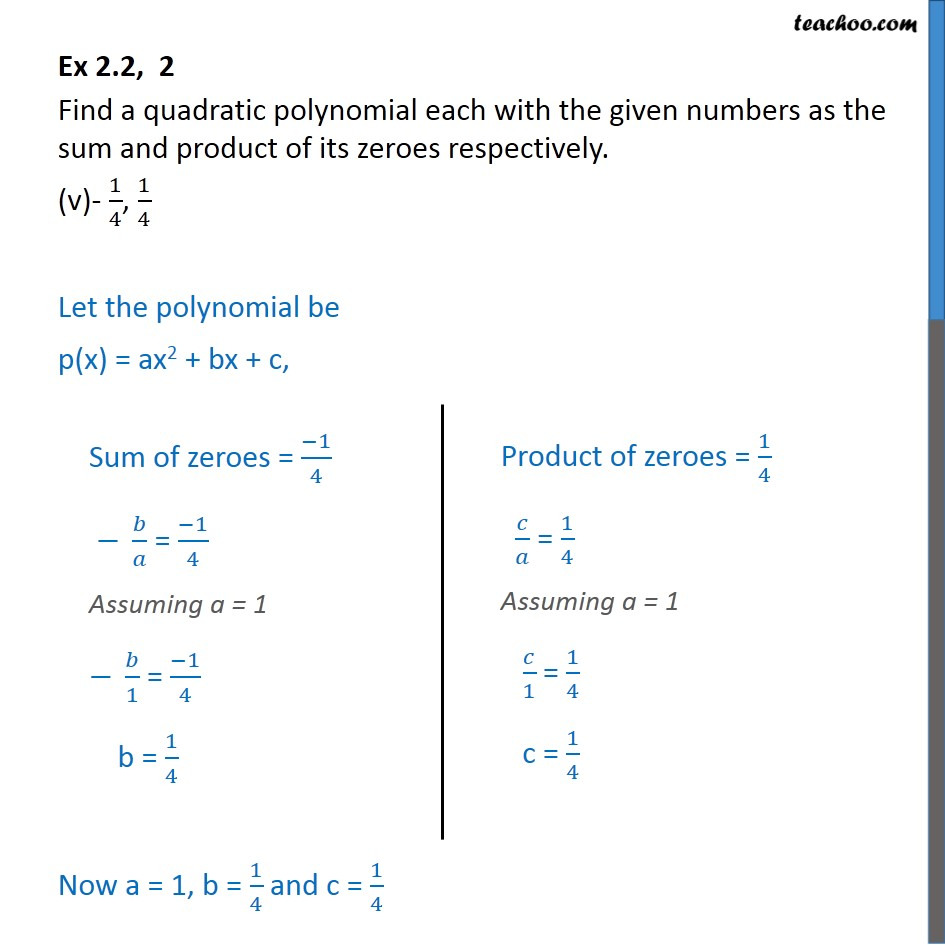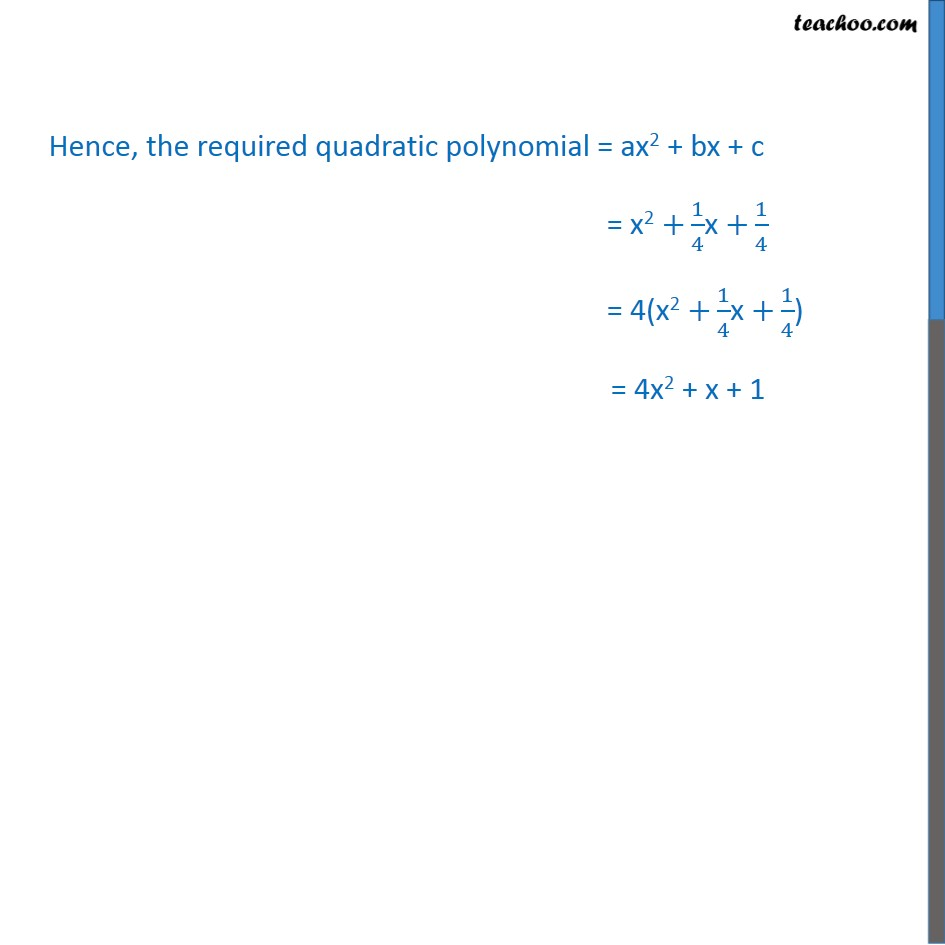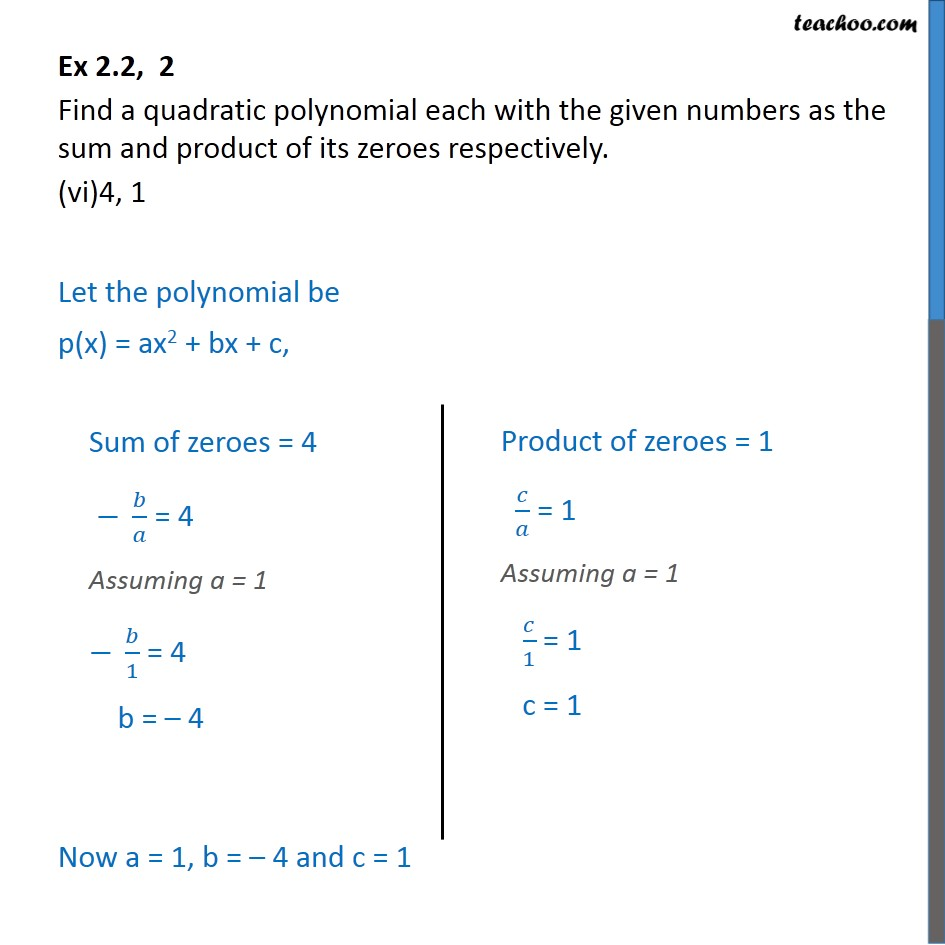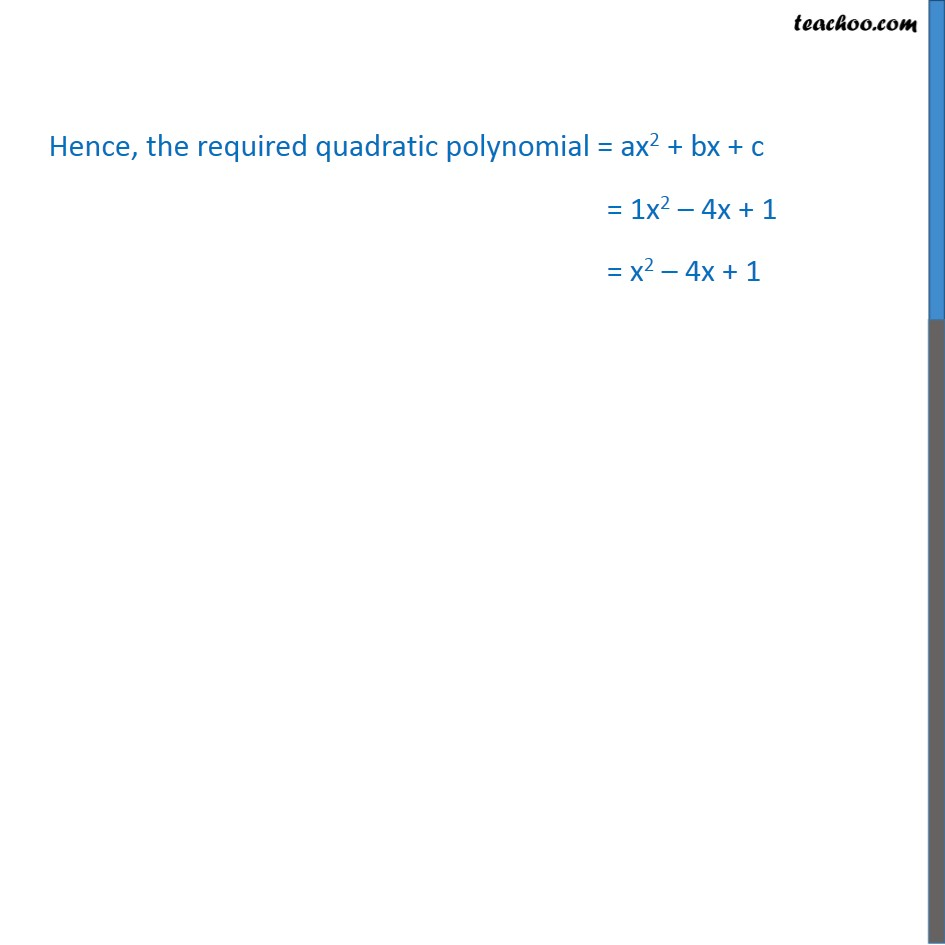1. Chapter 2 Class 10 Polynomials
2. Serial order wise
3. Ex 2.2

Transcript

Ex2.2, 2 Find a quadratic polynomial each with the given numbers as the sum and product of its zeroes respectively. (i) 1/4 , −1 Let the polynomial be p(x) = ax2 + bx + c, Now a = 1, b = – 1/4 and c = –1 Hence, the required quadratic polynomial = ax2 + bx + c = x2 −1/4x −1 = 4(x2 −1/4x −1) Ex 2.2, 2 Find a quadratic polynomial each with the given numbers as the sum and product of its zeroes respectively. (ii) √2 , 1/3 Let the polynomial be p(x) = ax2 + bx + c, Now a = 1, b = –√2 and c = 1/3 Hence, the required quadratic polynomial = ax2 + bx + c = x2 −√2x + 1/3 = 3(x2 −√2x + 1/3) Ex 2.2, 2 Find a quadratic polynomial each with the given numbers as the sum and product of its zeroes respectively. (iii) 0, √5 Let the polynomial be p(x) = ax2 + bx + c, Now a = 1, b = 0 and c = √5 Hence, the required quadratic polynomial = ax2 + bx + c = x2 + 0x + √5 = x2 + √5 Ex 2.2, 2 Find a quadratic polynomial each with the given numbers as the sum and product of its zeroes respectively. (iv)1,1 Let the polynomial be p(x) = ax2 + bx + c, Now a = 1, b = – 1 and c = 1 Hence, the required quadratic polynomial = ax2 + bx + c = 1x2 – 1x + 1 = x2 – x + 1 Ex 2.2, 2 Find a quadratic polynomial each with the given numbers as the sum and product of its zeroes respectively. (v)- 1/4, 1/4 Let the polynomial be p(x) = ax2 + bx + c, Now a = 1, b = 1/4 and c = 1/4 Hence, the required quadratic polynomial = ax2 + bx + c = x2 +1/4x +1/4 = 4(x2 +1/4x +1/4) Ex 2.2, 2 Find a quadratic polynomial each with the given numbers as the sum and product of its zeroes respectively. (vi)4, 1 Let the polynomial be p(x) = ax2 + bx + c, Now a = 1, b = – 4 and c = 1 Hence, the required quadratic polynomial = ax2 + bx + c = 1x2 – 4x + 1 = x2 – 4x + 1

Ex 2.2

Chapter 2 Class 10 Polynomials
Serial order wise

About the AuthorDavneet Singh
Davneet Singh is a graduate from Indian Institute of Technology, Kanpur. He has been teaching from the past 9 years. He provides courses for Maths and Science at Teachoo.